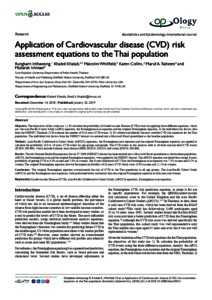# Application of Cardiovascular disease (CVD) risk assessment equations to the Thai population

KHATAB, Khaled, INTHAWONG, Rungkarn, WHITFIELD, Malcolm, COLLINS, Karen, RAHEEM, Maruf A and ISMAIL, Mubarak (2019). Application of Cardiovascular disease (CVD) risk assessment equations to the Thai population. Biostatistics and Epidemiology International Journal.Preview
PDF
Application of Cardiovascular diseasebeij_00013_25_01_019.pdf - Published Version

Official URL: http://ologyjournals.com/beij/
Related URLs:

## Abstract

Objective: The objectives of this study are: 1.) To calculate the probability of Cardiovascular Disease (CVD) events by applying three different equations, which are: the Asia-Pacific Cohort Study (APCS) equation, the Framingham-Asia equation and the original Framingham equation, to the individual risk factors data from the NHESIV, Thailand. 2) To estimate the number of 8-10 years CVD events. 3) To validate and identify the most suitable CVD risk equations for the Thai population. The individual risk factors from the NHESIV dataset was entered into a Microsoft Excel spreadsheet as the baseline population. Methods: Asia-Pacific Collaborative Cohort Study (APCCS) equations, the Framingham-Asia equation and the original Framingham equation, are applied to calculate the probability of 8 to 10 years CVD events by age groups and gender. The CVD events in this analysis refer to all fatal and non-fatal CVD events (ICD10, I00-I99), which include Ischemic heart disease (IHD) (ICD10, I20-I25) and stroke (ICD10, I60-I69). Results: The 4th National Health Examination Survey IV 2009 (NHESIV) dataset has been entered into a Microsoft Excel spreadsheet as the baseline population. APCCS, the Framingham-Asia and the original Framingham equations, were applied to the NHESIV dataset. The APCCS equation calculated the average 8-years probability of getting CVD as 8.3% in men and 7.8% in women. The 8-year likelihood of CVD in the Framingham-Asia equation was 7.2% in men and 8.1% in women. The original Framingham equation showed the highest probability of 10-years CVD which were 18.8% in men and 11.1% in women. Conclusions: The original Framingham equation overestimated the risk of CVD in the Thai population in all age groups. The Asia-Pacific Cohort Study (APCCS) and the Framingham-Asia equations, both performed better estimation than the original Framingham equation in both men and women.

Item Type: Article Symplectic Elements Symplectic Elements 04 Feb 2019 11:35 18 Mar 2021 02:03 http://shura.shu.ac.uk/id/eprint/23940View Item